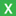Swap operations of the NBKR from 2000 to June 24, 2013Swap operations of the NBKR since June 25, 2013

### Swap operations of NBKR

Search the period (maximal period – 1 year):
--

 Date Swap operations of NBKR On the action base On the fix rate Direct swap Reverse swap Direct swap Reverse swap Volume (thou. soms) Volume (thou. US dollars) Weighted av. rate (%) Weighted term (days) Volume (thou. soms) Volume (thou. US dollars) Weighted av. rate (%) Weighted term (days) Volume (thou. soms) Volume (thou. US dollars) Fix rate (%) Weighted term (days) Volume (thou. soms) Volume (thou. US dollars) Fix rate (%) Weighted term (days)
!
!